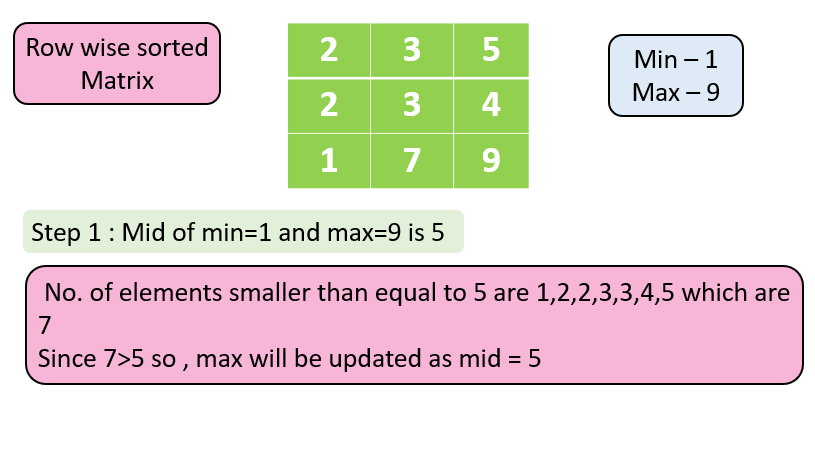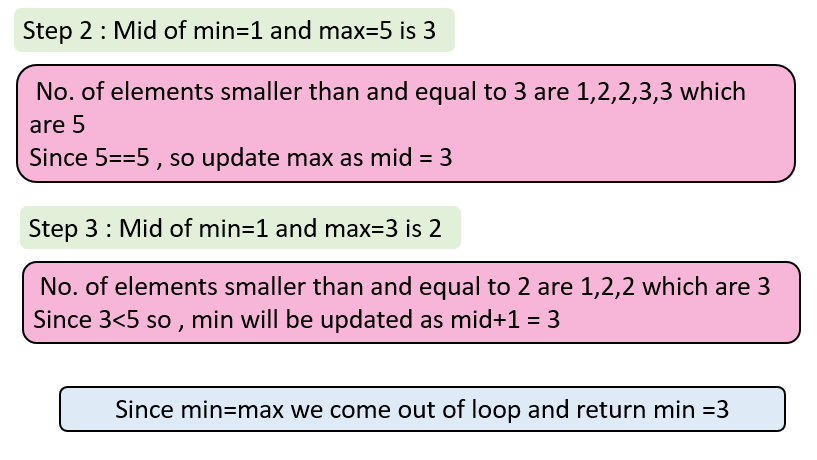# Median of Row Wise Sorted Matrix

In this article we will solve the most asked coding interview problem: Median of Row Wise Sorted Matrix

Problem Statement: Given a row-wise sorted matrix of size r*c, where r is no. of rows and c is no. of columns, find the median in the given matrix.

Assume – r*c is odd

Examples:

```Example 1:
Input:
r = 3 , c = 3
1 4 9
2 5 6
3 8 7
Output: Median = 5
Explanation: If we find the linear sorted array, the array becomes 1 2 3 4 5 6 7 8 9
So, median = 5

Example 2:
Input:
r = 3 , c = 3
1 3 8
2 3 4
1 2 5
Output: Median = 3
Explanation: If we find the linear sorted array, the array becomes 1 1 2 2 3 3 4 5 7 8
So, median = 3
```

### Solution

Disclaimer: Don’t jump directly to the solution, try it out yourself first.

Solution 1:

Approach 1: Brute Force Approach

The approach is very simple, just fill all elements in a linear array sort the array using the sort function, and then find the middle value (Median)

For Eg,

For the given matrix

1 3 8

2 3 4

1 2 5

We find the sorted linear array as 1 1 2 2 3 3 4 5 8

Now, the middle element of the array is 3.

Code:

## C++ Code

``````#include <bits/stdc++.h>
using namespace std;
// Function to find median  of row wise sorted matrix
int Findmedian(int arr, int row, int col)
{
int median[row * col];
int index = 0;
for (int i = 0; i < row; i++)
{
for (int j = 0; j < col; j++)
{
median[index] = arr[i][j];
index++;
}
}

return median[(row * col) / 2];
}
int main()
{
int row = 3, col = 3;
int arr = {{1, 3, 8},
{2, 3, 4},
{1, 2, 5}};
cout <<"The median of the row-wise sorted matrix is: "<<Findmedian
(arr, row, col) << endl;
return 0;
}
``````

Output: The median of the row-wise sorted matrix is: 3

Time Complexity: O(row*col log(row*col)) for sorting the array where r*c denotes the number of elements in the linear array.

Space Complexity: O(row*col)  for storing elements in the linear array

## Java Code

``````import java.util.*;
public class TUF {
// Function to find median of row wise sorted matrix
public static int Findmedian(int[][] arr, int row, int col) {
int[] median = new int[row * col];
int index = 0;
for (int i = 0; i < row; i++) {
for (int j = 0; j < col; j++) {
median[index] = arr[i][j];
index++;
}
}
return median[(row * col) / 2];
}
public static void main(String args[]) {
int row = 3, col = 3;
int[][] arr = {{1, 3, 8},
{2, 3, 4},
{1, 2, 5}};

System.out.println("The median of the row-wise sorted matrix is: "+
Findmedian(arr, row, col));

}
}
``````

Output: The median of the row-wise sorted matrix is: 3

Time Complexity: O(row*col log(row*col)) for sorting the array where r*c denotes the number of elements in the linear array.

Space Complexity: O(row*col)  for storing elements in the linear array

Approach 2: Efficient Approach (Using Binary Search)

Step 1: Find the minimum and maximum element in the given array. By just traversing the first column, we find the minimum element and by just traversing the last column, we find the maximum element.

Step 2: Now find the middle element of the array one by one and check in the matrix how many elements are present in the matrix.

Three cases can occur:-

• If count ==  (r*c+1)/2 , so it may be the answer still we mark max as mid since we are not referring indices , we are referring the elements and 5 elements can be smaller than 6 also and 7 also , so to confirm we mark max as mid.
• If count<(r*c+1)/2 , we mark  min as mid+1 since curr element or elements before it cannot be the answer.
• If count>(r*c+1)/2 , we mark max as mid , since elements after this can only be the  answer now.

Let’s discuss the approach with an example

For eg, the given array isCode:

## C++ Code

``````#include <bits/stdc++.h>
using namespace std;
int countSmallerThanMid(vector<int> &row, int mid)
{
int l = 0, h = row.size() - 1;
while (l <= h)
{
int md = (l + h) >> 1;
if (row[md] <= mid)
{
l = md + 1;
}
else
{
h = md - 1;
}
}
return l;
}
int findMedian(vector<vector<int>> &A)
{
int low = 1;
int high = 1e9;
int n = A.size();
int m = A.size();
while (low <= high)
{
int mid = (low + high) >> 1;
int cnt = 0;
for (int i = 0; i < n; i++)
{
cnt += countSmallerThanMid(A[i], mid);
}
if (cnt <= (n * m) / 2)
low = mid + 1;
else
high = mid - 1;
}
return low;
}
int main()
{
int row = 3, col = 3;
vector<vector<int>> arr = {{1, 3, 8},
{2, 3, 4},
{1, 2, 5}};
cout <<"The median of the row-wise sorted matrix is: "<<findMedian(arr)<<endl;
return 0;
}
``````

Output: The median of the row-wise sorted matrix is: 3

Time Complexity: O(row*log col) since the upper bound function takes log c time.

Space Complexity: O(1) since no extra space is required.

## Java Code

``````public class Main {
public static int countSmallerThanMid(int[] A, int mid, int n) {
int l = 0, h = n - 1;
while (l <= h) {
int md = (l + h) >> 1;
if (A[md] <= mid) {
l = md + 1;
} else {
h = md - 1;
}
}
return l;
}
public static int findMedian(int[][] A, int row, int col) {
int low = 1;
int high = 1000000000;
int n = row;
int m = col;
while (low <= high) {
int mid = (low + high) >> 1;
int cnt = 0;
for (int i = 0; i < n; i++) {
cnt += countSmallerThanMid(A[i], mid, col);
}
if (cnt <= (n * m) / 2)
low = mid + 1;
else
high = mid - 1;
}
return low;
}
public static void main(String args[]) {
int row = 3, col = 3;
int[][] arr = {{1, 3, 8},
{2, 3, 4},
{1, 2, 5}};
System.out.println("The median of the row-wise sorted matrix is: "+
findMedian(arr, row, col));

}
}
``````

Output: The median of the row-wise sorted matrix is: 3

Time Complexity: O(row*log col) since the upper bound function takes log c time.

Space Complexity: O(1) since no extra space is required.

Special thanks to Gurmeet Singh for contributing to this article on takeUforward. If you also wish to share your knowledge with the takeUforward fam, please check out this article